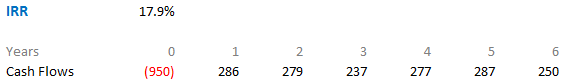Our journey to conquer the IRR ends here. Part 1 and Part 2 talk about what is IRR and its nuances and different workarounds

I am dedicating Part 3 to talk about Excel’s MIRR (modified internal rate of return) as a robust alternative to IRR. Given the fact that the IRR is not a robust metric, let’s see how can the MIRR function can do a much better job

### Quick Recap

I used the same example in Part 2The IRR is ~16.7% it assumes the cash flows are reinvested at 16.7% each year. Think about it practically, is it possible to reinvest each cash flow at such a high rate of return year on year? I guess not

And therefore the IRR over states the maximum possible return from the project

### Here comes the MIRR

Alright, lets pick up a set of cash flows. I am sure by now you must be so used to of seeing this. The IRR is around 18%On these cash flows lets calculate MIRR (Modified Internal Rate of Return).Notice the difference between MIRR (13.0%) and IRR (17.9%)Let me explain the formula

1. Values are nothing but the cash flows
2. Finance Rate is rate at which you borrow the money to be invested
• It is pretty simple to find the finance rate if you are raising the debt capital. It is rate of interest paid to the bank
• It can be quite a task to find an appropriate finance rate if you have a mix of Equity and Debt capital or only Equity capital. We aren’t getting into how to find the Finance Rate so lets assume something for now
3. Reinvestment Rate This was the primary reason we chose MIRR over IRR. Because we cannot reinvest the cash flows at IRR each year the MIRR functions asks for a more reasonable reinvestment rate for the cash flows

Notice the stark difference between the returns. The MIRR function gives a more reasonable return with more realistic assumptions. Mickinsey even wrote a white paper on why should you choose MIRR over IRR. A pretty interesting read

I am going to delve a bit deeper into how the IRR function works mathematically. So if you are good with just learning it on how to do in excel, I’ll make way for you..

But if you are curious then let’s dive in further. Don’t worry I am not that great at math.. I’ll make it really simple

### A manual way to calculate MIRR!

Thanks for staying tight. It would be nice to know how does the MIRR calculation work in a pen&paper way. Lets assume a little different set of cash flowsNotice that we have Initial 2 years of investment and the positive returns start coming in. I am going to do 2 things

1. Bring all cash outflows to present date by discounting them at a finance rate
2. Take all the cash inflows to future date by compounding them at a reinvestment rate

Something like thisAssume Finance Rate = 10 % and Reinvestment Rate = 8%

The discounting of investments (cash outflows) would look like thisThe above equation is equal to 777.27 (omit the negative sign)

And the compounding of earnings (cash inflows) would look like thisThe above equation is equal to 1561.81

### The MIRR Equation and Logic

Now that we have 2 critical things

1. A present day cash investment at a 10% finance rate i.e. 777.27
2. A future value of cash inflows at a more realistic 8% i.e. 1561.81

The MIRR answer the following question: At what rate the money grows if 777.27 becomes 1561.81 in 6 years?

The answer can be solved by this equation= 12.33%

Even if you tried to solve that by MIRR formula it would be the same### What are your views on IRR ?

Do you use IRR often? What are the challenges that you have faced while using IRR? Write to me about your experience